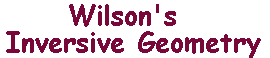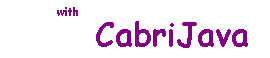The extended plane and i-lines.

 Suppose that C is a circle with centre O. We noted earlier that there is no inverse of O with respect to C, and that there is no point P with inverse O. Definition Let E be the euclidean plane. The extended plane E+ consists of the points of E, together with the point at infinity, ∞ Then, for circle D with centre Q, we extend iD to E+ by defining iD(Q) = ∞, and iD(∞) = Q. The extended map (which still has order 2) is also denoted by iD, and is still called inversion in D. Now suppose that L is a line in E. Then reflection in L is defined for all points of E, so the only way to extend the map to E+ is to map ∞ to itself. We refer to the extended map as inversion in L, and denote it by iL. If L is a line on E, then we define the extended line L+ to be the line L together with ∞. Motivation For P in E, P is on L if and only if iL(P)=P. Since ∞ also has this property, we regard it as "on" L In Apollonian Families, we discovered that each apollonian curve was either a circle or a line. We introduce a term to include both types in the extended plane. Definition A subset of E+ is an i-line if it is a circle or an extended line. Basic facts Suppose that M and N are i-lines. Then M is an extended line if and only if ∞ lies on M. The map iM is defined on E+, and is of order 2. A point P in E+ lies on M if and only if iM(P) = P. If M and N are extended lines, they meet at ∞ (and possibly once more). M and N may meet in up to two points - see the CabriJava pane. Any three distinct points in E+ determine a unique i-line. Only the last of these needs much justification. Suppose that the three points lie in E. Then either they are collinear (so define only a line), or they determine a unique circle (the circumcircle). Drag A or B to vary the circles, or drag the line. Watch the intersections change.

These i-lines are the "lines" of inversive geometry, and the inversions are the fundamental transformations.